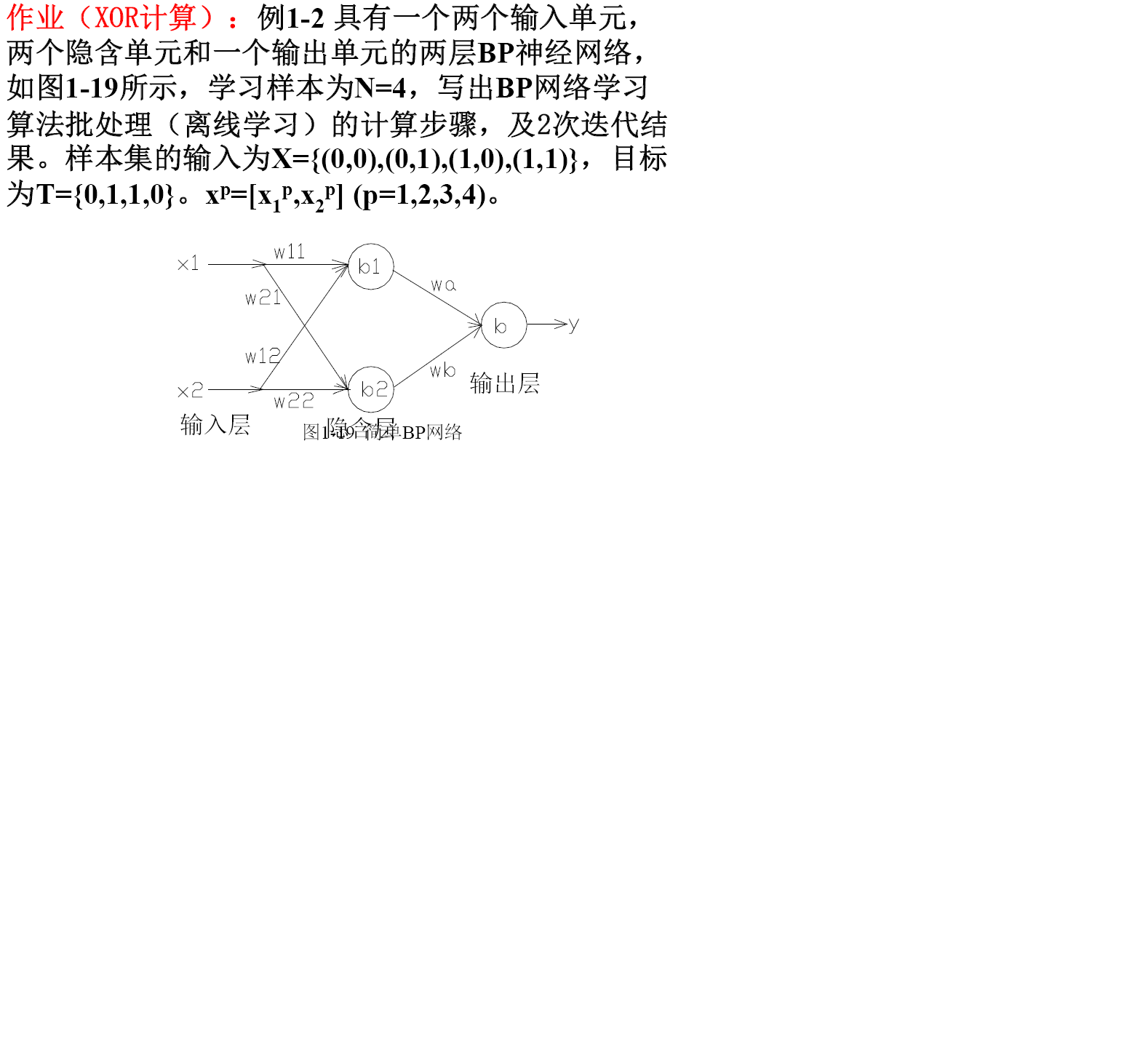matlab 解决神经网络

%traingd
clear;
clc;
P=[0 0;0 1;1 0;1 1];
T=[0 1 1 0];
%利用minmax函数求输入样本范围
net = newff(minmax(P),[2,1],{'tansig','purelin'},'trainrp');

net.trainParam.show=50;%
net.trainParam.lr=0.05;
net.trainParam.epochs=2;
net.trainParam.goal=1e-5;
[net,tr]=train(net,P,T);

net.iw{0,0}%隐层权值
net.b{0}%隐层阈值

net.lw{0,1}%输出层权值
net.b{1}%输出层阈值

sim(net,P)